##### The Japanese Maple specialist
Direct order Contact Help / Services Newsletter# Shelf to displaySearch

Japanese MaplesBonsai soil and fertiliserAcer seeds and more Young maples and bonsaiHostasWind bells and chimesSaxifragesVarieties introduced into Europe

Packing and shipping charges

# Izu no odoriko

Acer palmatum izu no odoriko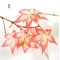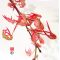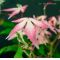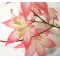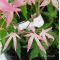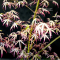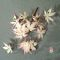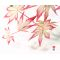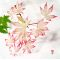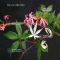ref. : 485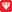Choose the right size
Pot size

This item is temporarily unavailable

###### Description

In one word: superb!
Unique in Europe!
Foliage of small green size of white, of rose. Some leaves are pink of other cream-colored white, absolutely splendid cultivar but difficult has multiply. Loses his/her/its leaves at the end of summer. Extremely rare variety in culture for aware collector.
Indeed this variety only possesses little chlorophyll and require that one keeps for a good vigor a part of his/her/its door transplants in leaf green.  Our expert's advice will allow you to succeed his culture. The delivered plant will be of small size + - 15/20 cm of height non branched out for 1.5 liter pot annd 20/40 cm for 3 liter pots. Be careful trees potted in spring 2019 don't touch the root ball before spring 2020.

#culture 2.7 #amoenum 2.7 #odoriko 2.7 #variety 2.7 #spring 2.6 #leaves 2.6 #green 2.5 #liter 2.5 #small 2.5 #white 2.5

###### Technical description
 Species Acer amoenum same species items Variety Acer palmatum izu no odoriko Leaf colour Variegated leaves same colour items Leaf shape Palmated same shaped items Adult height Less than 2 meters same height items Port Natural same port items Expositions Partial shade same exposition items

Formule
(( ROUND((CHAR_LENGTH(b.article_nom)-CHAR_LENGTH(REPLACE(b.article_nom, 'odoriko', '')))/LENGTH('odoriko')) + ROUND((CHAR_LENGTH(b.article_description)-CHAR_LENGTH(REPLACE(b.article_description, 'odoriko', '')))/LENGTH('odoriko')) ) * 2.7) + (( ROUND((CHAR_LENGTH(b.article_nom)-CHAR_LENGTH(REPLACE(b.article_nom, 'variety', '')))/LENGTH('variety')) + ROUND((CHAR_LENGTH(b.article_description)-CHAR_LENGTH(REPLACE(b.article_description, 'variety', '')))/LENGTH('variety')) ) * 2.7) + (( ROUND((CHAR_LENGTH(b.article_nom)-CHAR_LENGTH(REPLACE(b.article_nom, 'culture', '')))/LENGTH('culture')) + ROUND((CHAR_LENGTH(b.article_description)-CHAR_LENGTH(REPLACE(b.article_description, 'culture', '')))/LENGTH('culture')) ) * 2.7) + (( ROUND((CHAR_LENGTH(b.article_nom)-CHAR_LENGTH(REPLACE(b.article_nom, 'spring', '')))/LENGTH('spring')) + ROUND((CHAR_LENGTH(b.article_description)-CHAR_LENGTH(REPLACE(b.article_description, 'spring', '')))/LENGTH('spring')) ) * 2.6) + (( ROUND((CHAR_LENGTH(b.article_nom)-CHAR_LENGTH(REPLACE(b.article_nom, 'leaves', '')))/LENGTH('leaves')) + ROUND((CHAR_LENGTH(b.article_description)-CHAR_LENGTH(REPLACE(b.article_description, 'leaves', '')))/LENGTH('leaves')) ) * 2.6) + (( ROUND((CHAR_LENGTH(b.article_nom)-CHAR_LENGTH(REPLACE(b.article_nom, 'green', '')))/LENGTH('green')) + ROUND((CHAR_LENGTH(b.article_description)-CHAR_LENGTH(REPLACE(b.article_description, 'green', '')))/LENGTH('green')) ) * 2.5) + (( ROUND((CHAR_LENGTH(b.article_nom)-CHAR_LENGTH(REPLACE(b.article_nom, 'white', '')))/LENGTH('white')) + ROUND((CHAR_LENGTH(b.article_description)-CHAR_LENGTH(REPLACE(b.article_description, 'white', '')))/LENGTH('white')) ) * 2.5) + (( ROUND((CHAR_LENGTH(b.article_nom)-CHAR_LENGTH(REPLACE(b.article_nom, 'small', '')))/LENGTH('small')) + ROUND((CHAR_LENGTH(b.article_description)-CHAR_LENGTH(REPLACE(b.article_description, 'small', '')))/LENGTH('small')) ) * 2.5) + (( ROUND((CHAR_LENGTH(b.article_nom)-CHAR_LENGTH(REPLACE(b.article_nom, 'liter', '')))/LENGTH('liter')) + ROUND((CHAR_LENGTH(b.article_description)-CHAR_LENGTH(REPLACE(b.article_description, 'liter', '')))/LENGTH('liter')) ) * 2.5) + (( ROUND((CHAR_LENGTH(b.article_nom)-CHAR_LENGTH(REPLACE(b.article_nom, 'will', '')))/LENGTH('will')) + ROUND((CHAR_LENGTH(b.article_description)-CHAR_LENGTH(REPLACE(b.article_description, 'will', '')))/LENGTH('will')) ) * 2.4)

## Secure payment## Delivery

Our logistic partners :